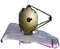# JWST ETC Integrated Emission Line SNRSignal-to-noise ratios (SNRs) are reported on a per-pixel basis in the JWST ETC. This page presents two methods for calculating the integrated SNR of an emission line.

The JWST Exposure Time Calculator (ETC) reports the signal-to-noise ratio (SNR) per pixel in the 1D Plots, Reports, and in the SNR column in the Calculations box. There is currently no functionality in the ETC to calculate the SNR across a resolved emission line. Until such a feature is added, integrated line SNRs must be calculated using the ETC Downloads available for each calculation.

# Method 1: Recommended option

The recommended procedure for calculating the SNR for a resolved emission line is as follows:

2. Open/read the "lineplot_extracted_flux.fits" and "lineplot_extracted_noise.fits" files in your preferred coding language.
3. Subtract the continuum from the extracted flux and propagate the errors according to the continuum subtraction method. If you chose the No Continuum option in the original calculation, skip this step.
4. To compute the integrated SNR: Sum the continuum-subtracted extracted flux values across the emission feature, then divide by the uncertainties added in quadrature.

# Method 2: Less accurate option

A slightly simpler, less accurate option assumes the uncertainties are constant across the emission feature: